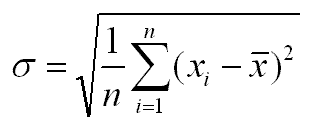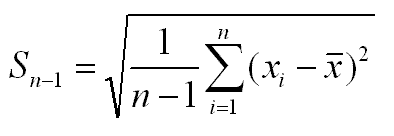The fomula for standard deviation is as below:And the sample standard deviation is calculated as:n is the number of samples

x is the mean value of the samples

xi is the value of the ith sample